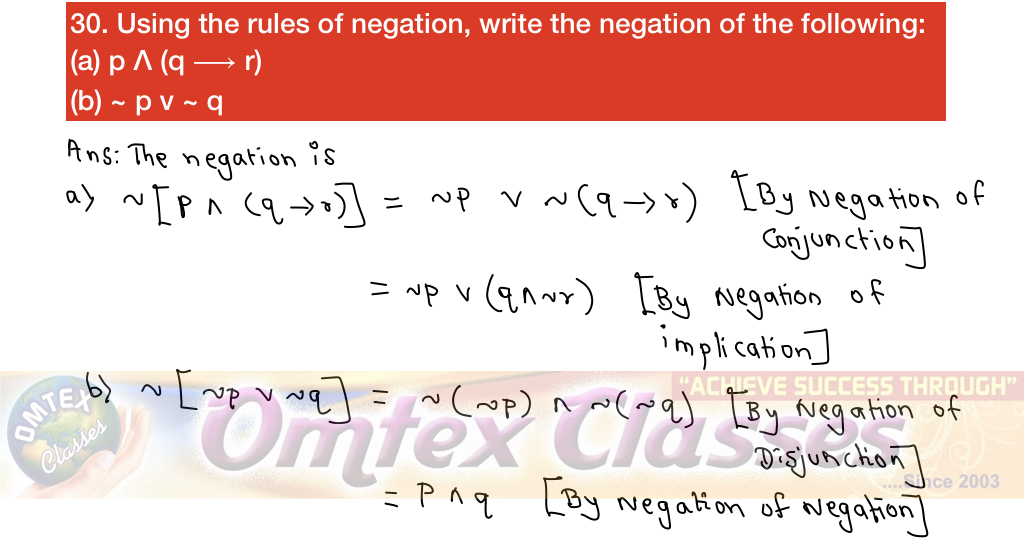### Logic Important Question for HSC Maths I Board Exam 2019

Logic Important Question for HSC Maths I Board Exam 2019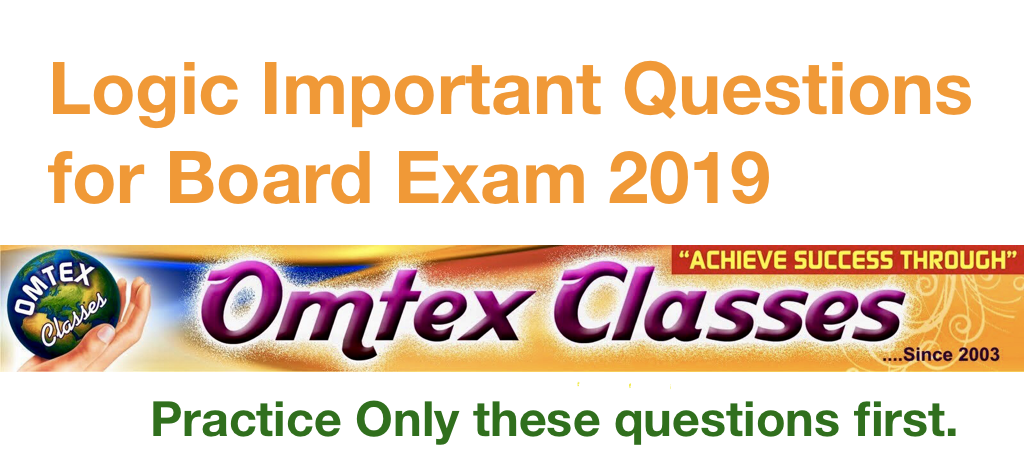1. Draw Venn diagram for the truth of the following statements:
(a) All rational numbers are real numbers.
(b) Some rectangles are squares.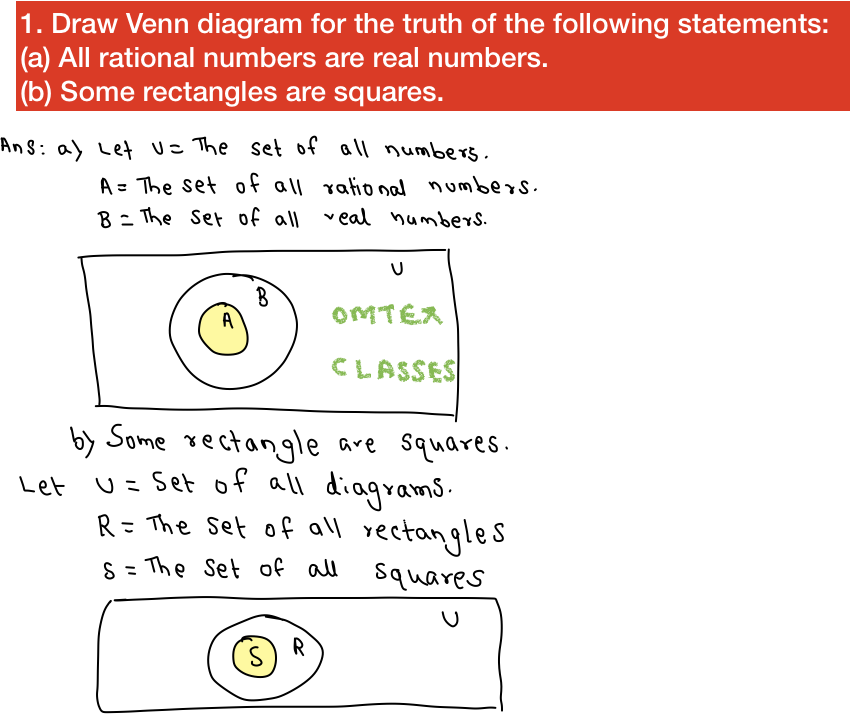2. Examine whether the following statement pattern is tautology, contradiction or contingency: p v ~ (p Λ q)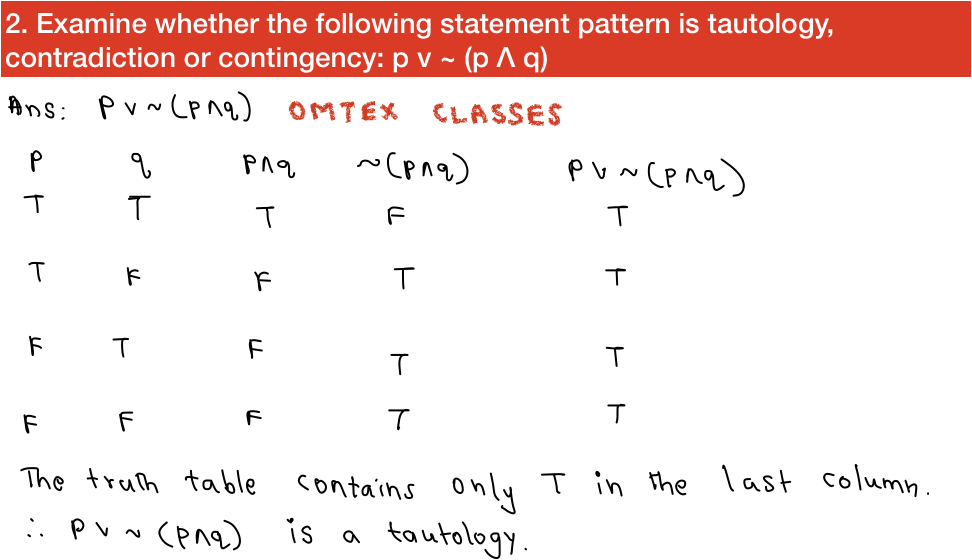3. Using the truth table show that ~(p ⟶ ~ q) = p Λ q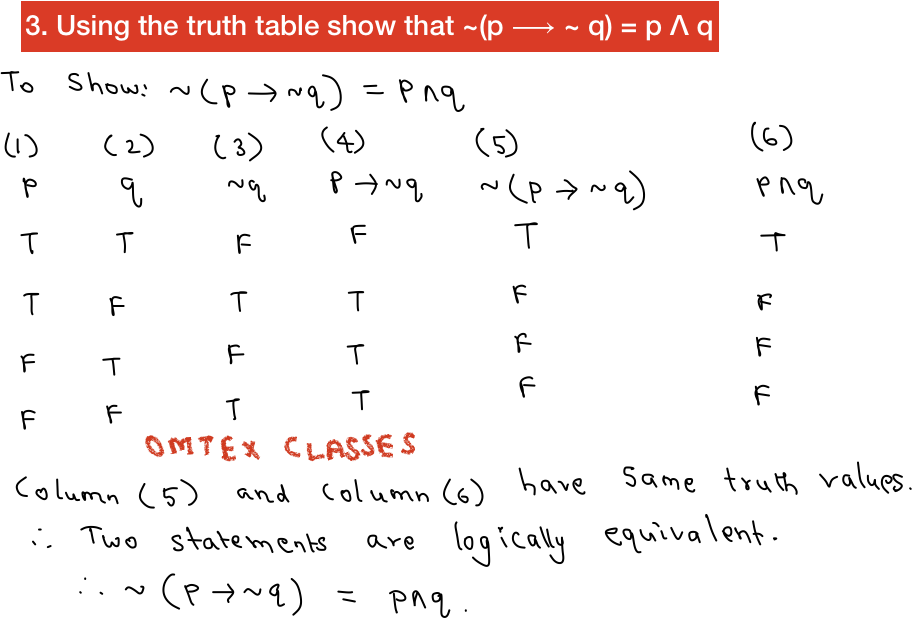4. Write the negation of the following statements:
(a) Radha likes tea or coffee.
(b) ∃x ∈R such that x + 3 ≥ 10.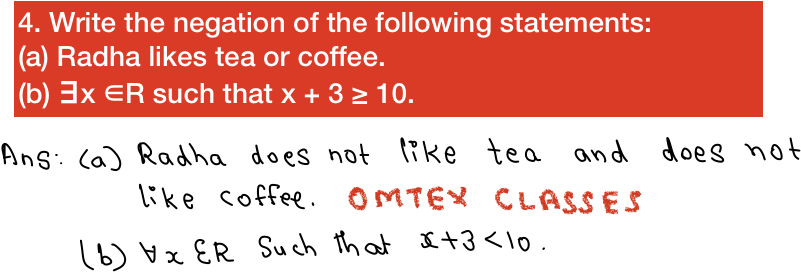5. If p : It is raining.
q: It is humid.
Write the following statements in symbolic form.
(a) It is raining or humid.
(b) It is raining then it is humid.
(c) It is raining but not humid.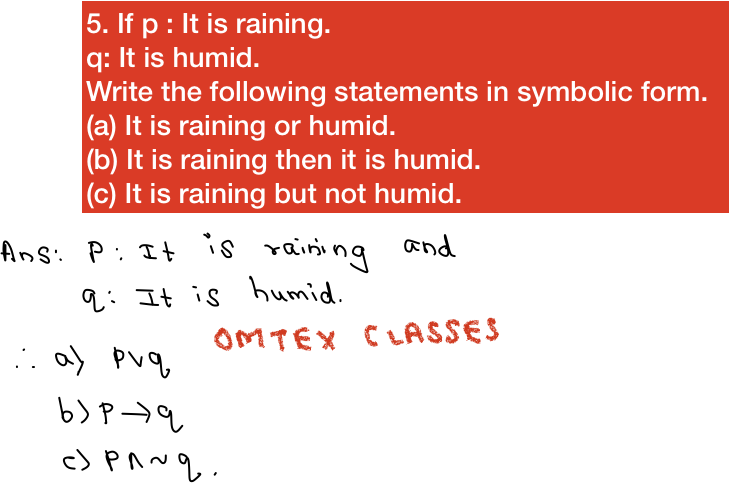6. Using truth table, examine whether the following statement pattern is tautology, contradiction or contingency: p v ~(p Λ q)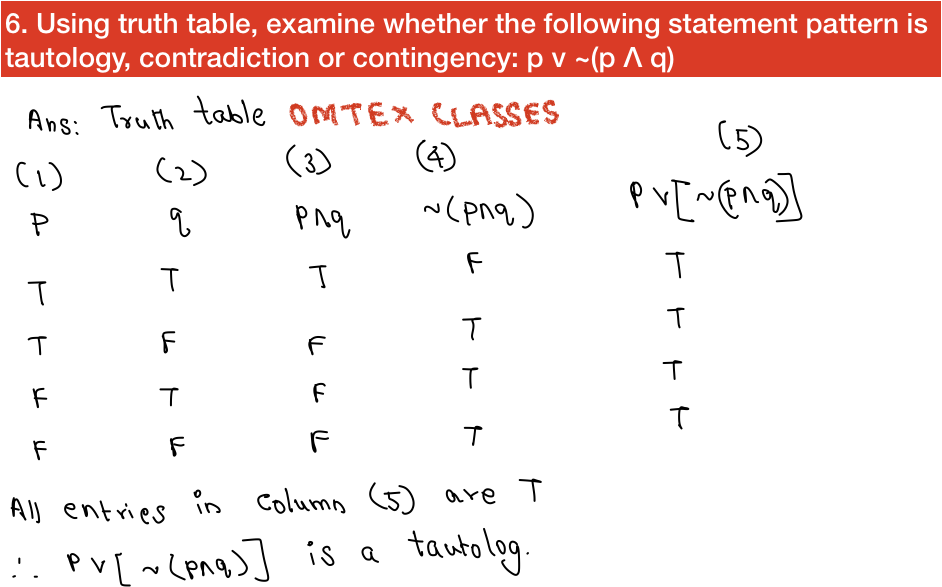7. Express the truth of the following statements with the help of Venn diagram.
(a) No circles are polygon.
(b) If a quadrilateral is rhombus, then it is a parallelogram.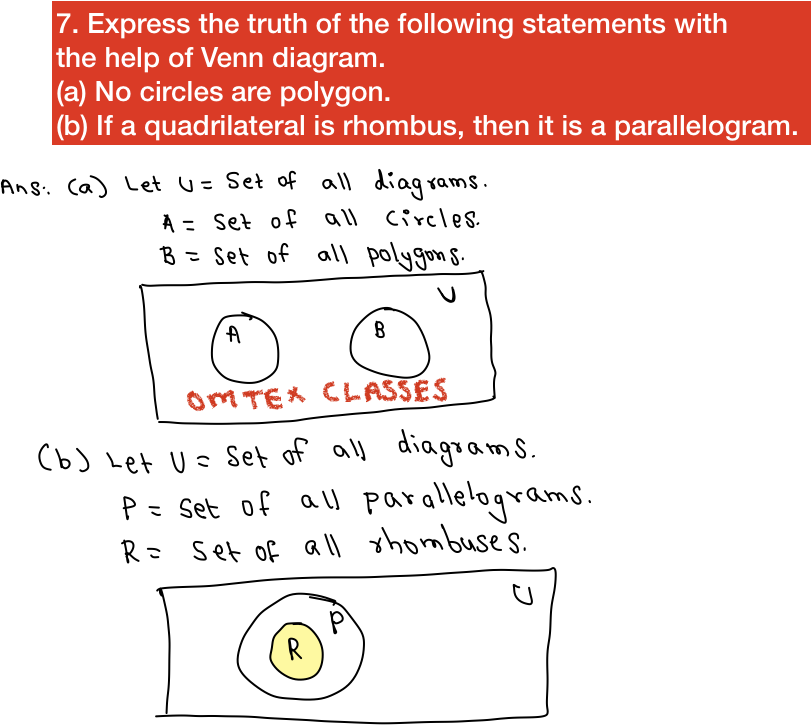8. Write the negation of the following statements:
(a) The number 6 is an even number or the number 25 is a perfect square.
(b) If  x ∈ A ⋂ B, then x ∈ A and x ∈ B.9. Using the truth table verify that
p v (q Λ r) = (p v q) Λ (p v r)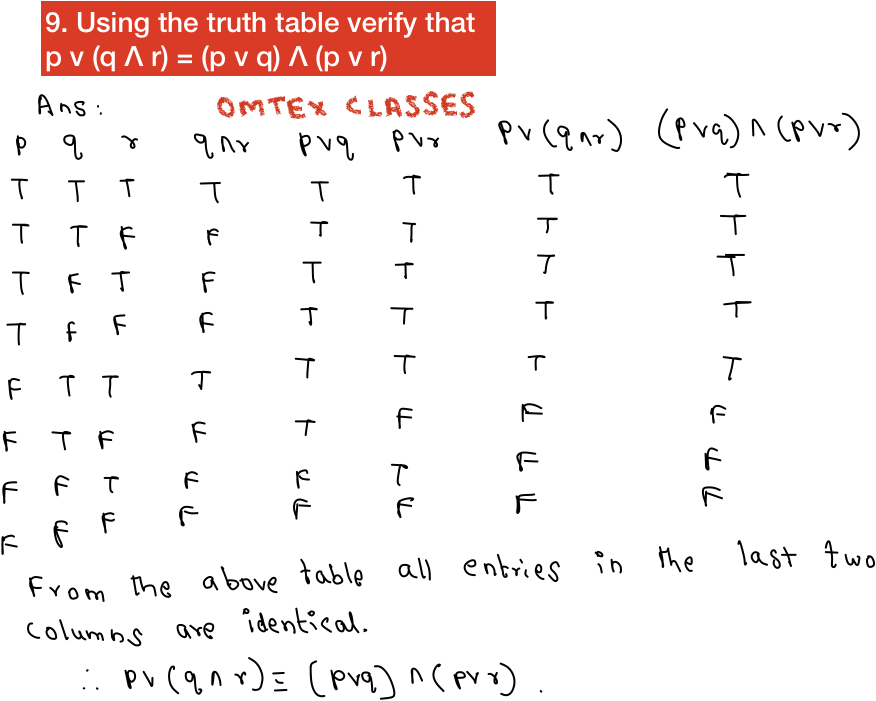10. Write the truth value of the negation of each of the following statements:
(a) The Sun sets in the East.
(b) cos2 Θ + sin2 Θ = 1, for all Θ ∈ R.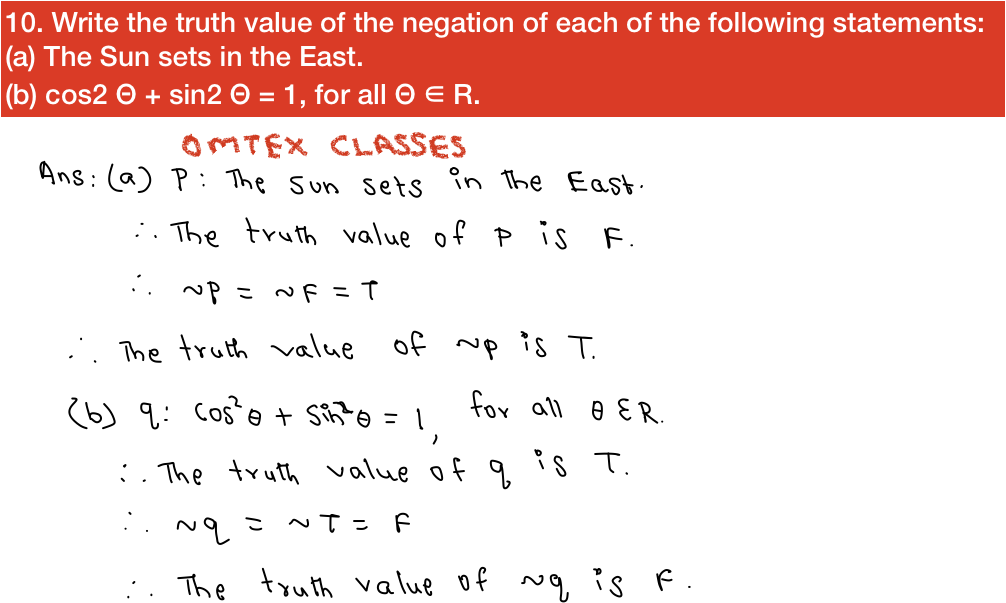11. Show that the following statement pattern in contingency: (~p v q)  ⟶ [p Λ (q v ~q)]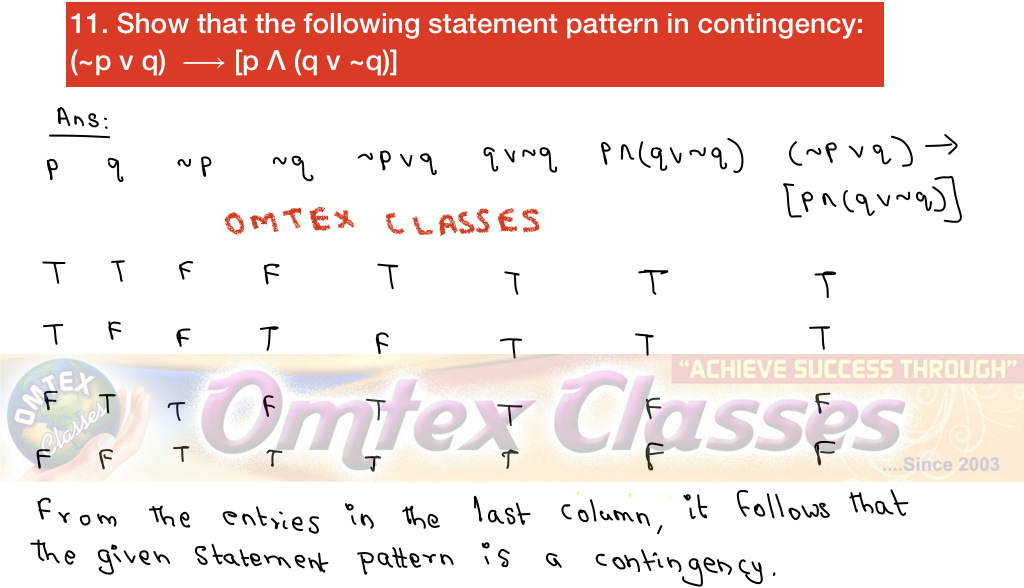12. Using the Venn diagram, examine the logical equivalence of the following statements:
(a) Some politicians are actors. 1
(b) There are politicians who are actors.
(c) There are politicians who are not actors.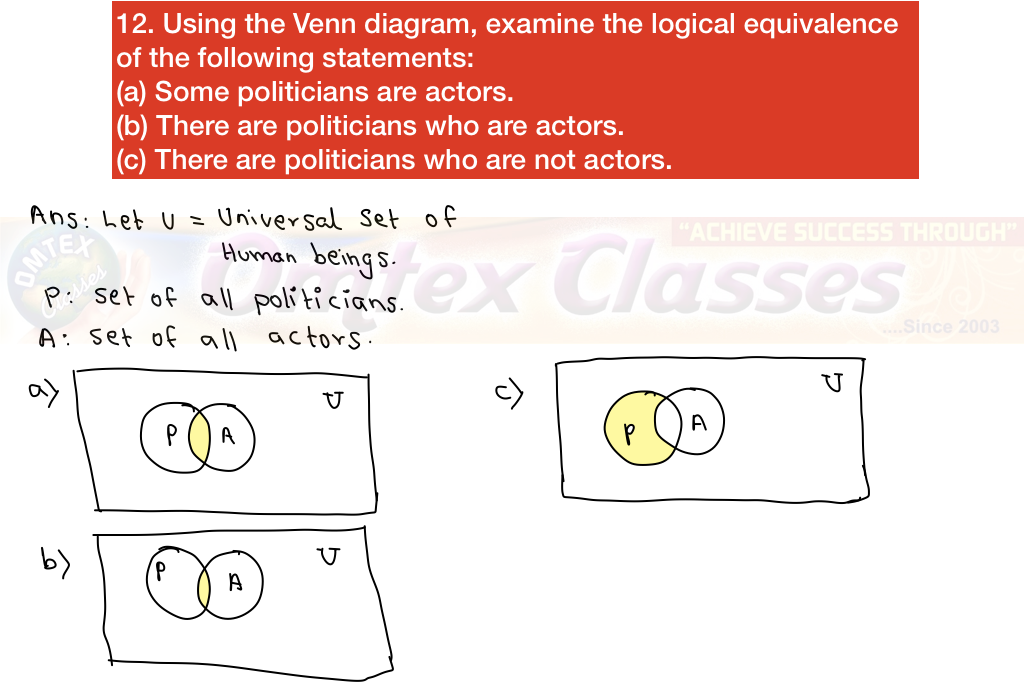13. Write the negation of the following statements.
(a) ∀y ∈ N, y2 + 3 ≤ 7
(b) If the lines are parallel then their slopes are equal.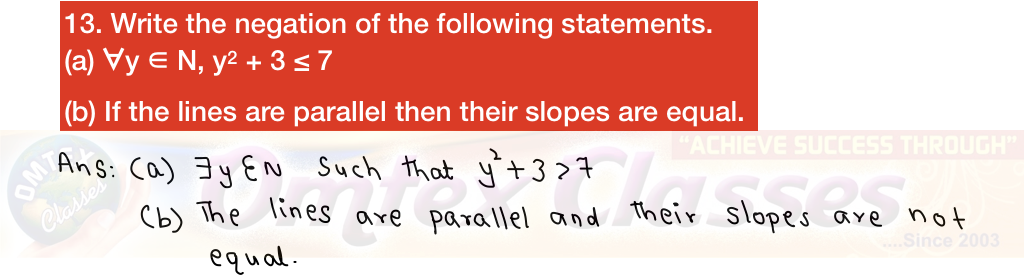14. State which of the following sentences are statements. In case of statement, write down the truth value:
(a) Every quadratic equation has only real roots.
(b) √-4 is a rational number.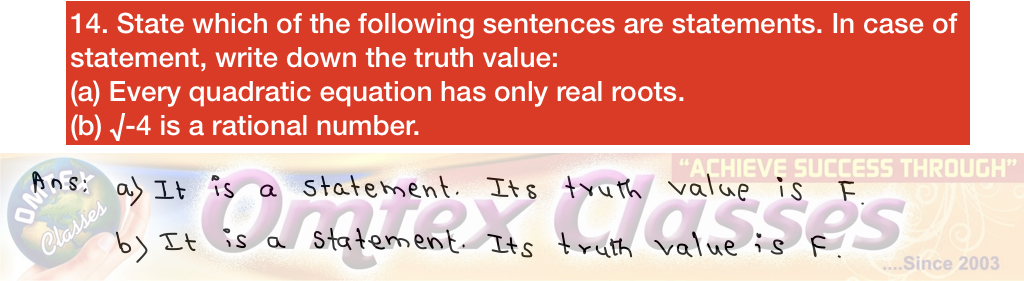15. Express the truth of each of the following statements using Venn diagram.
(1) All teachers are scholars and scholars are teachers.
(2) If a quadrilateral is a rhombus then it is a parallelogram.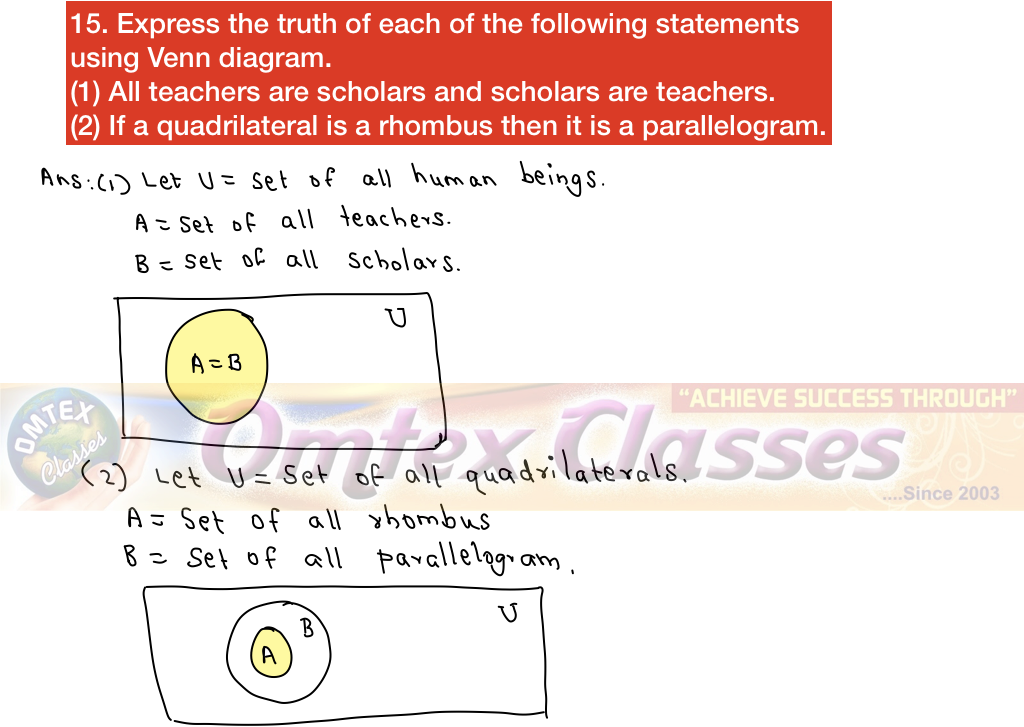16. Write converse and inverse of the following statement:
"If Ravi is good in logic then Ravi is good in Mathematics."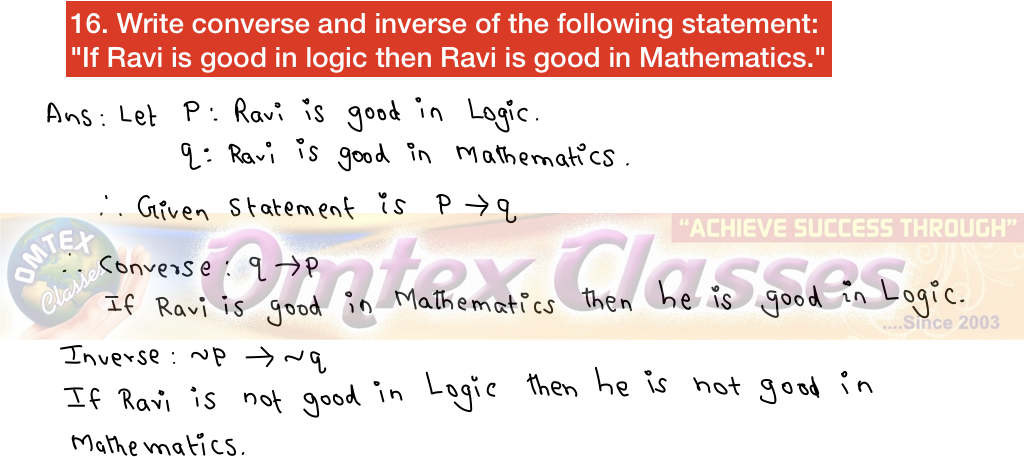17. Use the quantifiers to convert each of the following open sentences defined on N into true statement:
(a) 5x - 3 < 10
(b) x2 ≥ 1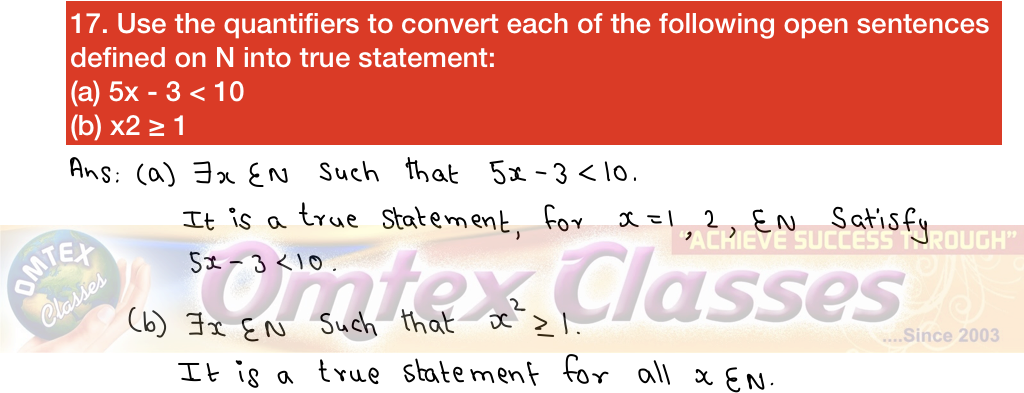18. Write converse, inverse and contrapositive of the statement. "If two triangles are not congruent then their areas are not equal".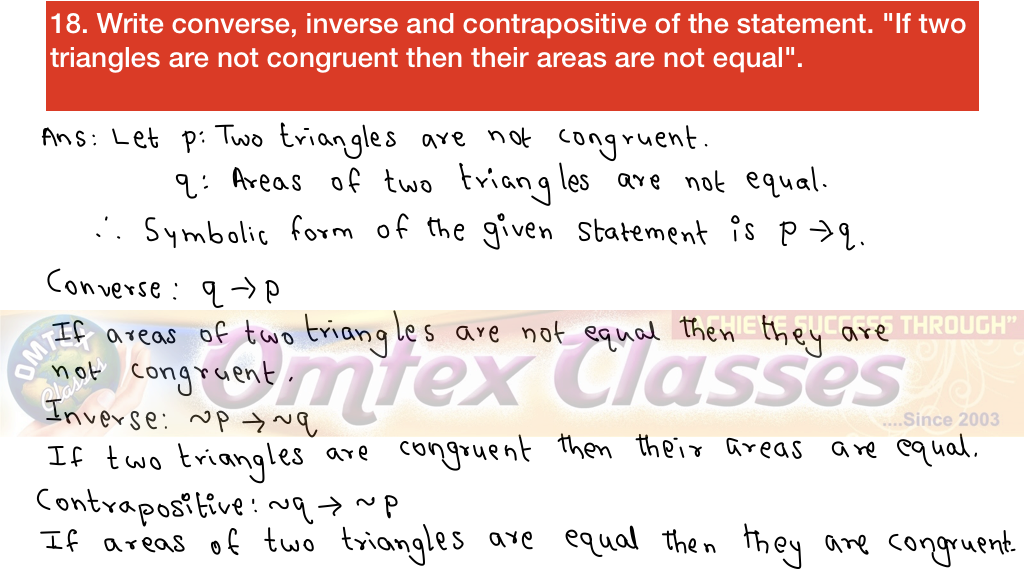19. By Constructing the truth table, determine whether the following statement pattern is a tautology, contradiction or contingency. (p ⟶ q) Λ (p Λ ~q).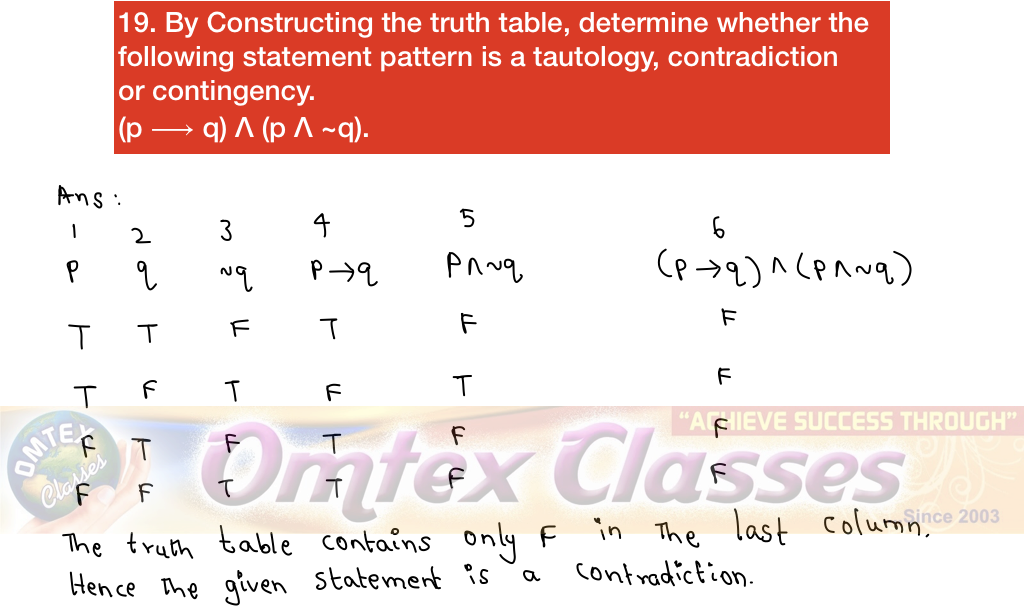20. Express the following statement in symbolic form and write its truth value.
"If 4 is an odd number, then 6 is divisible by 3."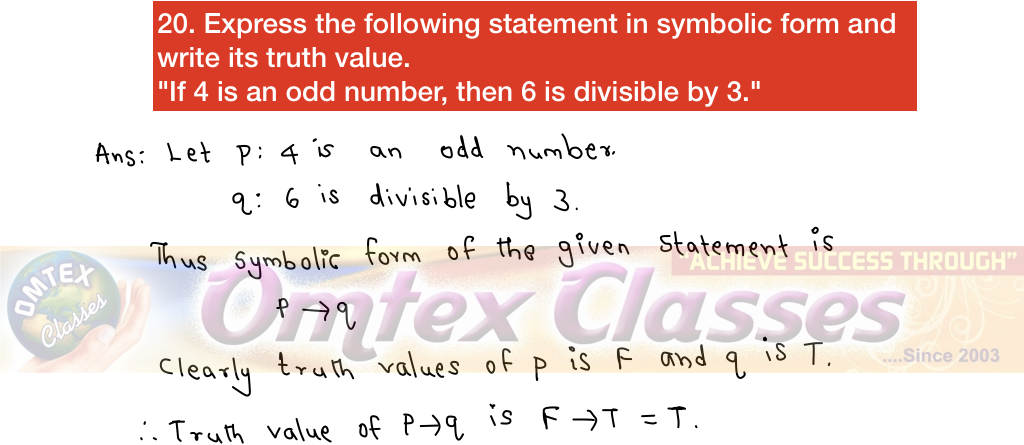21. Prove that the following statement pattern is equivalent: (p v q) ⟶ r and (p ⟶ r) Λ (q ⟶ r)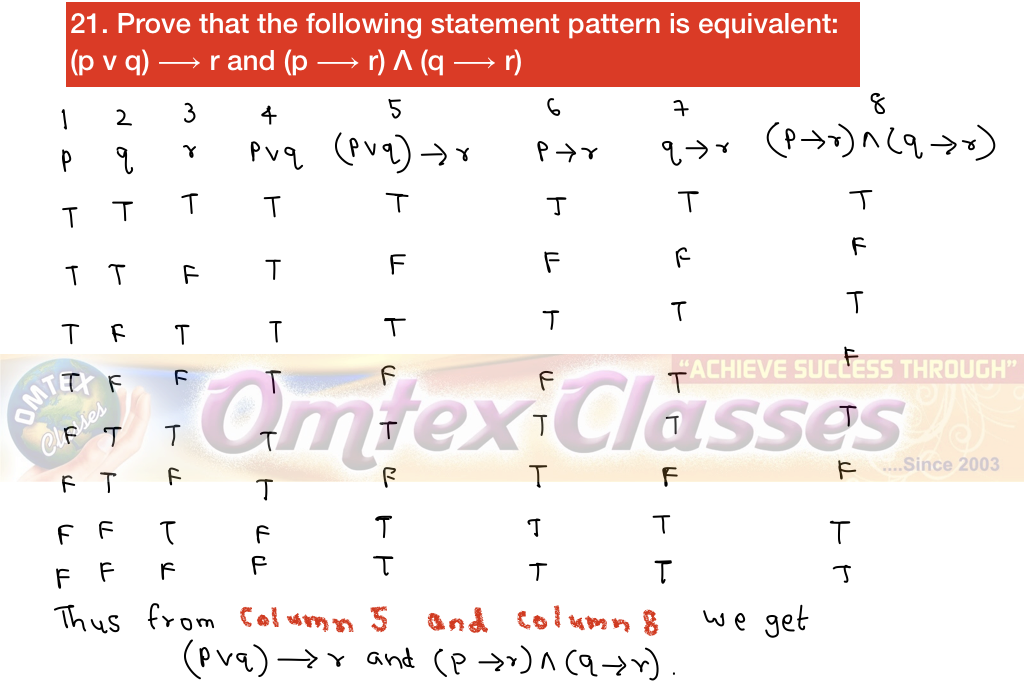22. Express the truth of each of the following statements by Venn diagram.
(a) Some hardworking students are obedient.
(b) No circles are polygons.
(c) All teachers are scholars and scholars are teachers.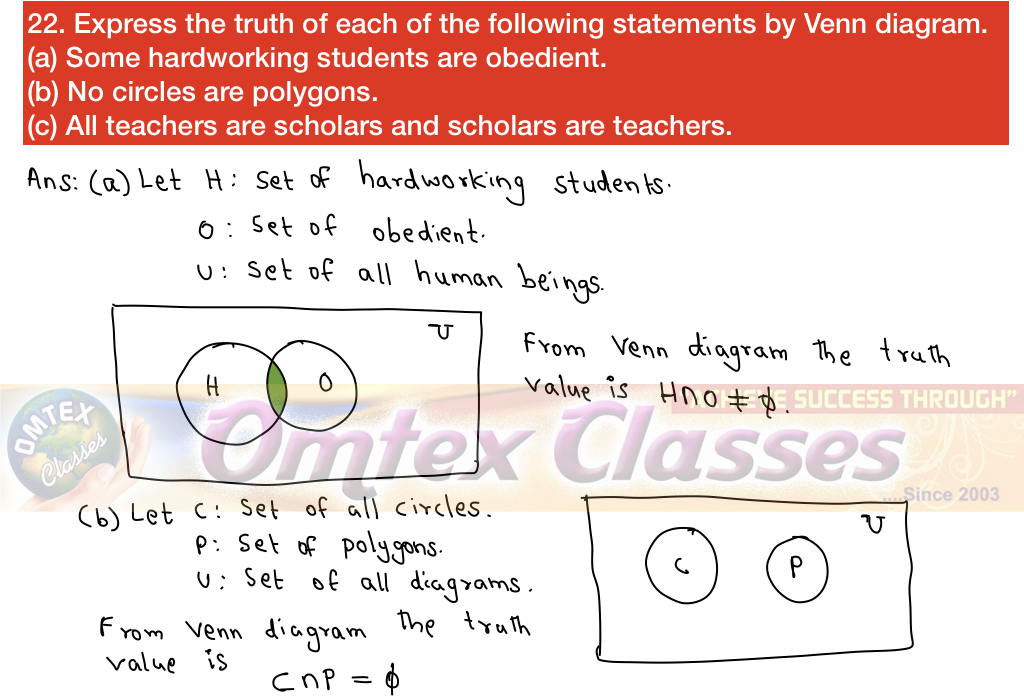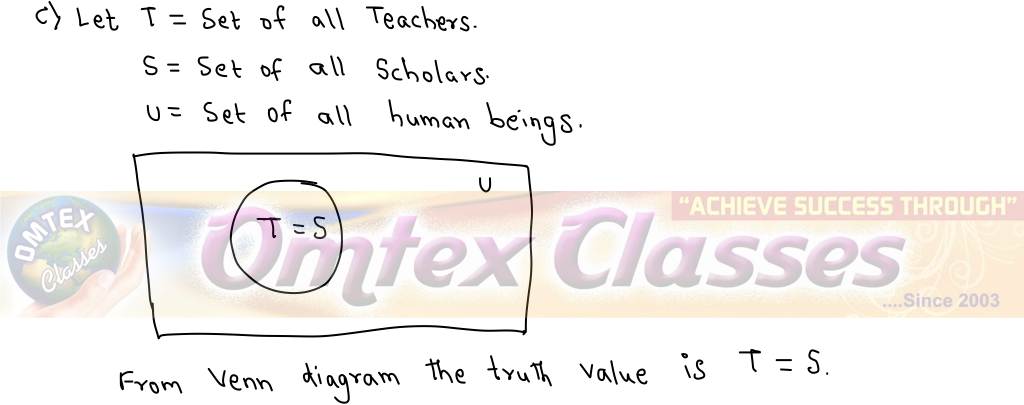23. Write the following statements in symbolic form.
(a) Either 49 is a perfect square or 39 is divisible by 11.
(b) it is not true that if 'i' is a real number, then '2' is an even prime number.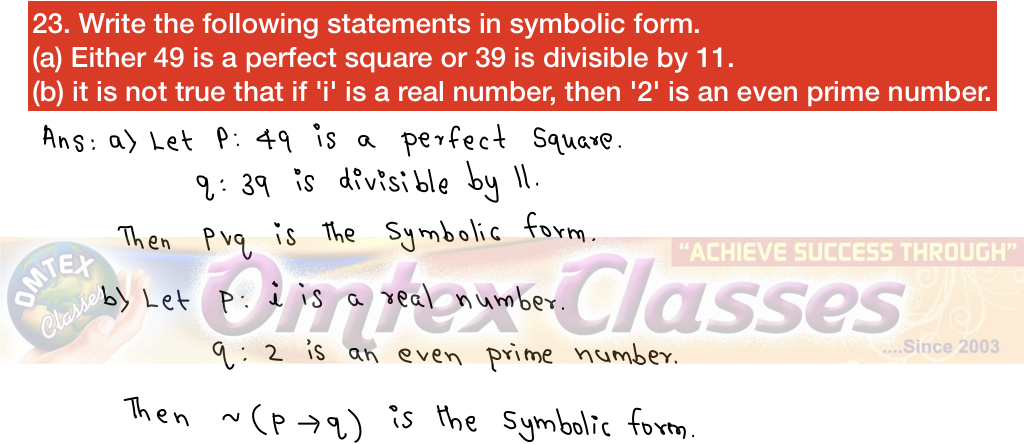24. Examine whether the following statement (p Λ q) v (~ p v ~ q) is a tautology or contradiction or neither of them.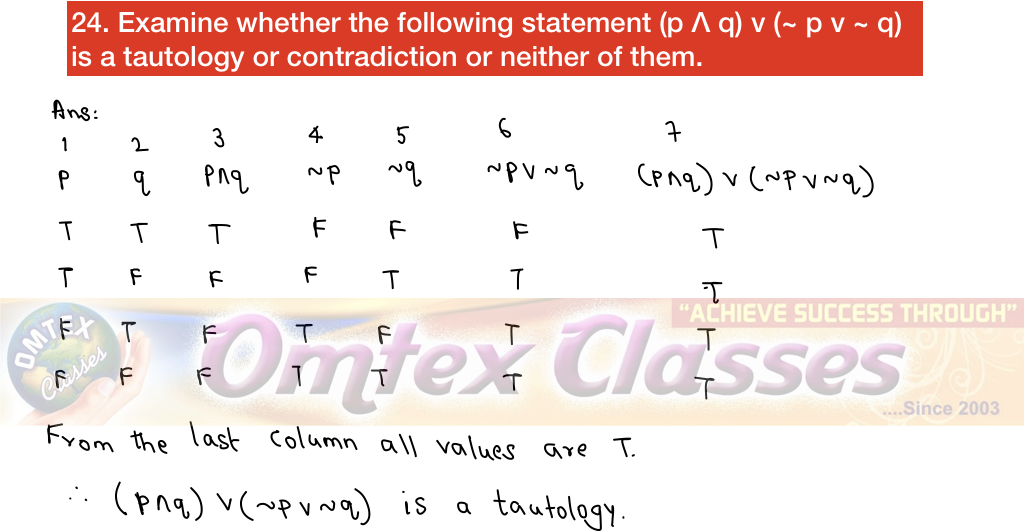25. Write the negation of the following statements:
(a) Chetan has black hair and blue eyes.
(b) ∃x ∈R such that x2 + 3 > 0.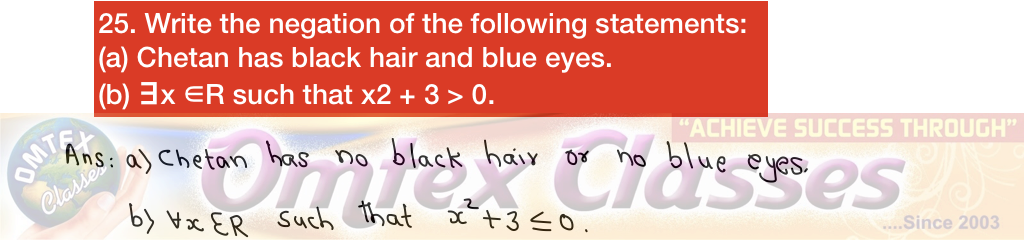26. If p: It is a day time.
q: It is warm.
Give the verbal statements for the following symbolic statements:
(a) p Λ ~ q
(b) p v q
(c) p ↔️q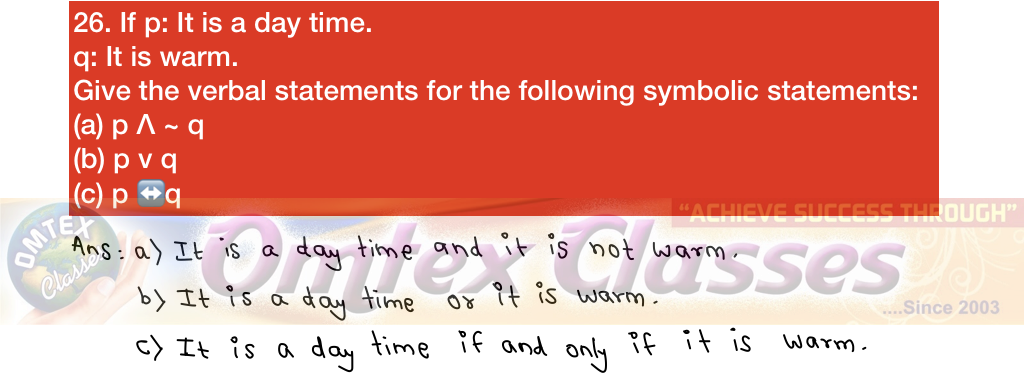27. Using the truth table, examine whether the statement pattern (p ⟶ q) ↔️(~ p v q) is a tautology, a contradiction or a contingency.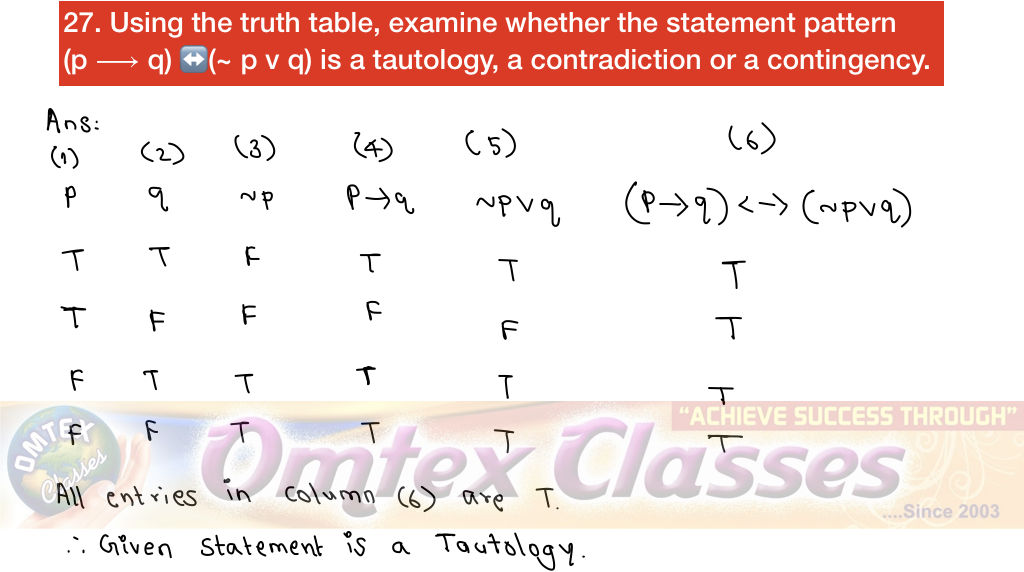28. If p : It is a day time
q: It is warm
Give the verbal statements for the following symbolic statements:
(a) p Λ ~ q
(b) p ⟶ q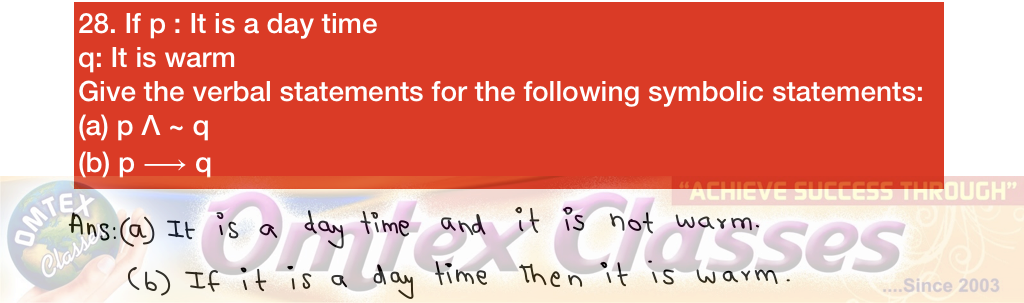29. Express the truth of each of the following statements using Venn diagrams.
(a) No circles are polygons.
(b) Some quadratic equations have equal roots.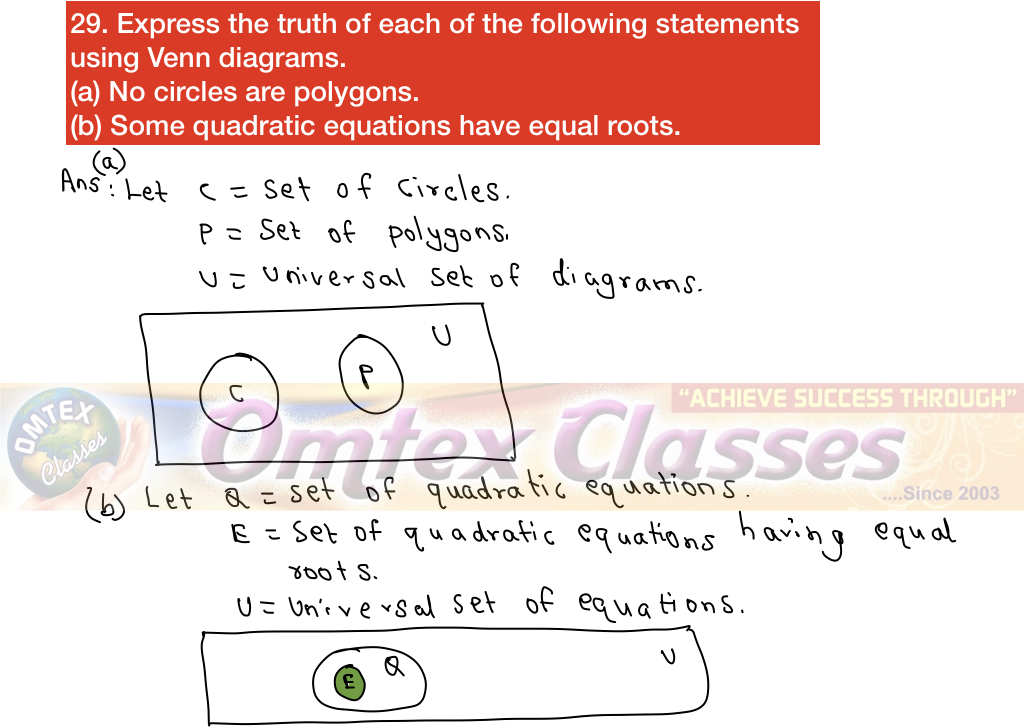30. Using the rules of negation, write the negation of the following:
(a) p Λ (q ⟶ r)
(b) ~ p v ~ q